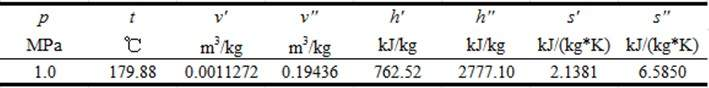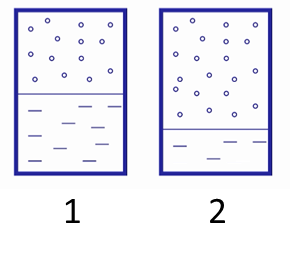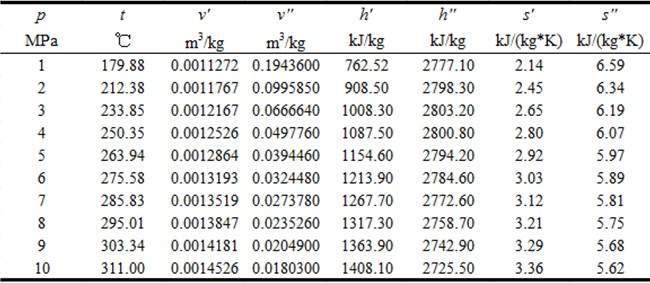# 第6章 水蒸气的性质与过程--第6章 章节小测验【含答案】 工程热力学

（1）单选题

1) 容器内气体的比容为______m3/kg;A  0.00112

B  0.00224

C  0.10327

D  0.19436

（2）单选题

（接上题）

2) 容器内蒸汽的干度是______；

A  0.00577

B  0.06332

C  0.5

（3）单选题

（接上题）

3) 容器的容积是______L。

A  2.24

B  25.44

C  194.36

（4）单选题

(已知p=3.8MPa,t=430℃时，h=3287.8 kJ/kg, s=6.9005 kJ/(kg*K)；p=6 kPa时，h’=151.48 kJ/kg, h”=2566.6 kJ/kg, s’=0.52082 kJ/(kg*K), s”=8.3290 kJ/(kg*K))

A  78.8%

B  82.8%

C  88.8%

（5）单选题

A  正确

B  错误

（6）单选题

A  正确

B  错误

（7）单选题

A  正确

B  错误

（8）单选题

A  正确

B  错误

（9）单选题

A  正确

B  错误

（10）单选题A  正确

B  错误

（11）单选题

A  正确

B  错误

（12）单选题

A  正确

B  错误

（13）单选题

A  正确

B  错误

（14）单选题

A  正确

B  错误

（15）单选题

1) p = 1 MPa，t = 100 ℃；A  未饱和水

B  饱和水

C  湿饱和蒸汽

D  干饱和蒸汽

E  过热蒸汽

（16）单选题

（接上题）

2)  p = 5 MPa，v = 0.04 m3/kg；

A  未饱和水

B  饱和水

C  湿饱和蒸汽

D  干饱和蒸汽

E  过热蒸汽

（17）单选题

（接上题）

3)  p = 9 MPa, s = 4 kJ/(kg*K)。

A  未饱和水

B  饱和水

C  湿饱和蒸汽

D  干饱和蒸汽

E  过热蒸汽